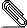# [HACKERS] Equivalence Rules

```Hi

My question is:
Does PostgreSQL implements equivalence rules(from those are listed in
email's attachment)?
Which function or which part of source code(in PostgreSQL ) implements
the equivalence rules?
I think, this should be implemented in query optimization part of
PostgreSQL, but which rule
and where, I don't know?
I want to use that(function or part of source code), to produce the
equivalence Relational Algebras (based on equivalence rules in
attachment) for a given SQL query(Relational Algebra).```
```
Thanks
```EquivalenceRules.pdf
```--